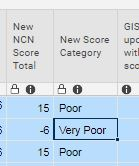# IF formula issue

The formula I've created isn't working correctly and I can't figure out what I'm doing wrong.

=IF([New NCN Score Total]120 = 15, IF([New NCN Score Total]120 <= 14, IF([New NCN Score Total]120 <= 9, IF([New NCN Score Total]120 <= 6, "Very Good"), "Good"), "Poor"), "Very Poor")

Scores

15 = Very Good

10 to 14 = Good

7 to 9 = Poor

(-)6 to 6 = Very Poor

Currently, when 15 is entered, it shows Poor which is not right at all. Can anyone help?

Thanks

FazTags:

• Try this

=IF([New NCN Score Total]@row >= 15, "Very Good", IF([New NCN Score Total]@row >= 10, "Good", IF([New NCN Score Total]@row >= 7, "Poor", IF([New NCN Score Total]@row < 7, "Very Poor"))))

When building a nested IF statement, you want it to return the string (Very Good) if it meets the logical expression.  So, if 15 is >= 15, say "Very Good".  If it does not, try the next logical expression, etc.

• That worked perfectly. Awesome, thanks so much.

## Help Article Resources

Want to practice working with formulas directly in Smartsheet?

Check out the Formula Handbook template!